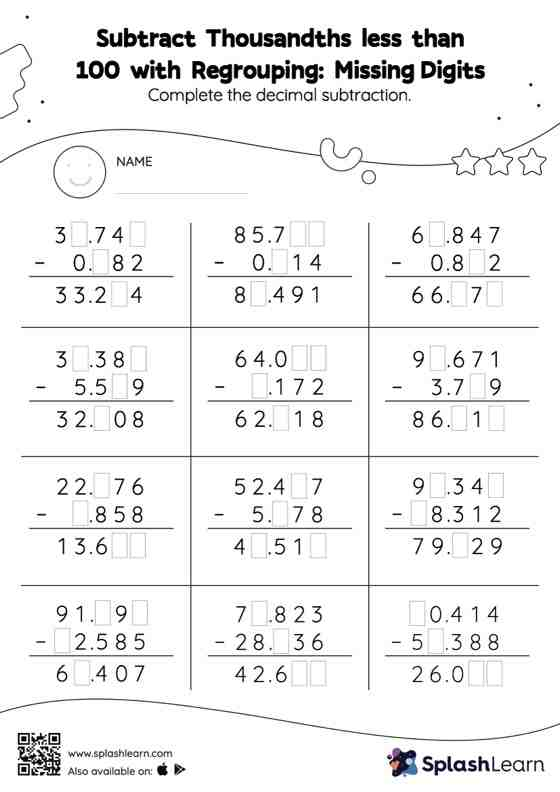# Subtract Thousandths less than 100 with Regrouping: Missing Digits Worksheet

Home > Subtract Thousandths less than 100 with Regrouping: Missing DigitsDoes your child know how to subtract thousandths less than 100 with regrouping? Invite them to practice this concept here. Students find the missing number while subtracting decimals by using the relationship between addition and subtraction. To reach the answer, they also use the relationship between ones, tenths, hundredths, thousandths, etc., To regroup the numbers in subtract thousandths less than 100 with regrouping worksheet. As the worksheet uses the column method, it is helpful in getting students toward higher accuracy, especially with bigger numbers and in scenarios where regrouping is required.